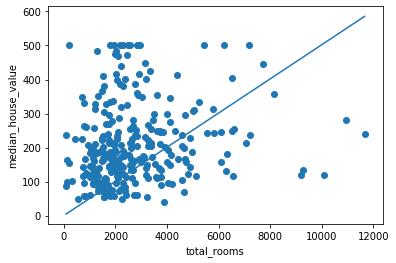# 机器学习-TensorFlow建模过程 Linear Regression线性拟合应用

2019/04/10 10:10

TensorFlow是咱们机器学习领域非常常用的一个组件，它在数据处理，模型建立，模型验证等等关于机器学习方面的领域都有很好的表现，前面的一节我已经简单介绍了一下TensorFlow里面基础的数据结构即：Tensor和Dataset； 这里咱们开始介绍TensorFlow的建模过程以及验证模型的一些简单方法。其实无论是sklearn还是TensorFlow，他们的模型建立过程都是相似的，都是经历columns类型声明，模型定义，数据训练，validation等等几个步骤。前面的几节内容我已经简单的介绍了如何用sklearn建立tree_based模型，这里我主要是想演示TensorFlow的应用，所以我就用linear regressor来当做例子来演示TensorFlow是如何从数据加载一直到数据验证的阶段。至于线性拟合的实现的具体细节，我在下一节的内容会从数学的角度具体解释的。本节内容所使用的数据都是来自于网络中，大家可以忽略具体的数据的意思，主要的了解TensorFlow的应用过程，不必过于纠结于模型的细节部分，模型的细节我会在随后的章节解释。好了，那么咱们现在开始吧

cali_housing_dataset_original = pd.read_csv("https://download.mlcc.google.com/mledu-datasets/california_housing_train.csv")
cali_housing_dataset_original["median_house_value"] /= 1000.0
#create a random generator
generator = np.random.Generator(np.random.PCG64())
#permutate the data frame
cali_housing_dataset_permutation = cali_housing_dataset_original.reindex(
generator.permutation(cali_housing_dataset_original.index)
)
cali_housing_dataset_permutation.describe()

#select the features that we will use in the model trainning process
my_feature = cali_housing_dataset_permutation[["total_rooms"]]
#select the targets of the dataset
targets = cali_housing_dataset_permutation[["median_house_value"]]

既然咱们的数据都准备好了，那么之后那么得定义一下咱们数据的类型（每一个column的类型都得定义），将来在咱们定义模型的时候咱们也得将columns的信息传递给咱们的模型对象；以及用什么optimizer将来来训练咱们的模型，这个optimizer将来也得传递给咱们的模型对象。 具体optimizer是什么我下面会慢慢讲的。为了方便演示，还是先上代码给大家看

#indicates what is the data type of the feature to tensorflow
feature_columns = [tf.feature_column.numeric_column("total_rooms")]
#using stochastic gradient descent as the optimizer for our model
#to ensure the magtitute of gradient do not become too large, we apply clipping_norm to our optimizer
my_optimizer = tf.optimizers.SGD(learning_rate = 0.0000001, clipnorm=5.0)

linear_regressor = tf.estimator.LinearRegressor(
feature_columns = feature_columns,
optimizer = my_optimizer
)

def my_input(features, targets, batch_size=500, shuffle=True, num_epochs=None):

"""
epochs: while trainning, in the case of steps is larger than the length of dataset, we set the epochs to None, which means repeat forever. However,
in trainning process, we have the steps to control the total number of iterations.  While in the case of making predictions of a given dataset, we must
set epochs to 1 and shuffle to False. Because we only want the input function return the dataset once, otherwise the function will keep returnning the
results forvere once and once again.

shuffle: in the trainning process, in order to balance the dataset, we set it to True. However while in prediction process, we have to set it to False, which
could help us to evaluate the outputs. For example, if the outputs are shuffled, we have no way to compare the outputs to our original lables.

"""

#convert panda dataframe to a dict of Numpy array
features = {key:tf.multiply(np.array(value),1) for key,value in dict(features).items()}
#construct a dataset
ds = tf.data.Dataset.from_tensor_slices((features,targets))
ds = ds.batch(batch_size).repeat(num_epochs)

if shuffle:
ds = ds.shuffle(buffer_size = 10000)
return ds

linear_regressor.train(
input_fn = lambda:my_input(my_feature, targets),
steps = 1000
)

#create a input function for prediction
prediction_input_fn = lambda:my_input(my_feature,targets,shuffle=False,num_epochs=1)
#prediction
predictions = linear_regressor.predict(input_fn = prediction_input_fn)
predictions = np.array([item["predictions"] for item in predictions])

#errors MSE
mean_squared_error(targets,predictions)

sample = cali_housing_dataset_permutation.sample(n=300)
x_0 = sample["total_rooms"].min()
x_1 = sample["total_rooms"].max()
linear_regressor.get_variable_names()#retrieve the name of the variable
weights = linear_regressor.get_variable_value("linear/linear_model/total_rooms/weights")#returns the value of variable given by name
bias = linear_regressor.get_variable_value("linear/linear_model/bias_weights")#retruns the value of bias
y_0 = weights*x_0+bias
y_1 = weights*x_1+bias
plt.plot([x_0,x_1],[y_0,y_1])#plot our regression line
plt.ylabel("median_house_value")#label the y Axes
plt.xlabel("total_rooms")#label the x Axes
plt.scatter(sample["total_rooms"],sample["median_house_value"])#plot a scatter plot from the sample
plt.show()可以看得出来，拟合的还不错。嘿嘿，关于模型训练过程的可视化，后面还有很多种，以后我慢慢说，例如：x坐标是steps, y坐标是loss, 也是非常常见的一种方式。

0
0 收藏

### 作者的其它热门文章0 评论
0 收藏
0